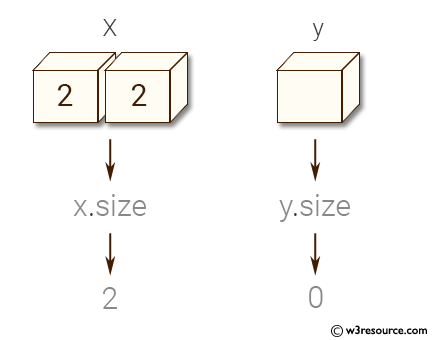﻿ NumPy: Check whether the numpy array is empty or not - w3resource# NumPy: Check whether the numpy array is empty or not

## NumPy: Array Object Exercise-95 with Solution

Write a NumPy program to check whether the numpy array is empty or not.

Pictorial Presentation:Sample Solution:

Python Code:

``````import numpy as np
x = np.array([2, 3])
y = np.array([])
# size 2, array is not empty
print(x.size)
# size 0, array is empty
print(y.size)
```
```

Sample Output:

```2
0
```

Python Code Editor:

Have another way to solve this solution? Contribute your code (and comments) through Disqus.

What is the difficulty level of this exercise?

Test your Python skills with w3resource's quiz

﻿

## Python: Tips of the Day

List comprehension:

```>>> m = [x ** 2 for x in range(5)]
>>> m
[0, 1, 4, 9, 16]
```